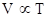Chapter 10.8, Problem 3.2ACP

Chapter
Section
Textbook Problem

You wish to fill a 75 L airbag at 25 °C to 3.0 atm using ammounium nitrate. a. What mass of NH4NO3 would be required? b. What are the partial pressures of N2O and H2O in the airbag?

(a)

Interpretation Introduction

Interpretation:

The required mass of NH4NO3 has to be calculated.

Concept Introduction:

Different laws have been put forward to introduce properties of gases:

Boyle’s law:

At fixed temperature, the volume of a fixed amount of gas is inversely proportional to the pressure exerted by the gas.

P1V(n,Twillbeconstant)

Charles’s law: States that volume is directly proportional to temperature when the gas is held at constant pressure and number of molecules.

VT

General Gas Law: Combining Charles’s law and Boyle’s law we get the General gas law or combined gas law.

P1V1T1=P2V2T2

• To relate the amount of gas and the volume.
• VnatconstantTandP

Combining all these together ideal gas law is introduced:

PV=nRT

Dalton’s law of partial pressure: The total pressure of gases in a mixture of gases is the sum of the pressure of each gas in the mixture

Mole fraction: Quantity which defines the number of moles of a substance in a mixture divided by the total number of moles of all substances present.

xa=nantotal

pa=xa×Ptotal

Partial pressure of a gas in the mixture of gases is the product of mole fraction of the gas and the total pressure

Explanation

The mass of NH4NO3 required to fill a 75L air bag at given conditions.

Ideal gas law: PV=nRT

R=0.0827L.atmK.molTshouldbeexpressedinKPshouldbeexpressedinatm

Given data:

P=3atmT=25°C=25+273=298KV=75L

From ideal gas equation:

n=PVRT=3atm×75L0.0827L.atm/K.mol×298K=9

(b)

Interpretation Introduction

Interpretation:

The partial pressures of N2OandH2O in the airbag has to be calculated.

Concept Introduction:

Different laws have been put forward to introduce properties of gases:

Boyle’s law:

At fixed temperature, the volume of a fixed amount of gas is inversely proportional to the pressure exerted by the gas.

P1V(n,Twillbeconstant)

Charles’s law: States that volume is directly proportional to temperature when the gas is held at constant pressure and number of molecules.General Gas Law: Combining Charles’s law and Boyle’s law we get the General gas law or combined gas law.

P1V1T1=P2V2T2

• To relate the amount of gas and the volume.
• VnatconstantTandP

Combining all these together ideal gas law is introduced:

PV=nRT

Dalton’s law of partial pressure: The total pressure of gases in a mixture of gases is the sum of the pressure of each gas in the mixture

Mole fraction: Quantity which defines the number of moles of a substance in a mixture divided by the total number of moles of all substances present.

xa=nantotal

pa=xa×Ptotal

Partial pressure of a gas in the mixture of gases is the product of mole fraction of the gas and the total pressure

Still sussing out bartleby?

Check out a sample textbook solution.

See a sample solution

The Solution to Your Study Problems

Bartleby provides explanations to thousands of textbook problems written by our experts, many with advanced degrees!

Get Started